Python73

• 基本的数据类型与结构
• 基本的逻辑控制语句

# 开发环境

1. 解释器： Python 3.8.8 | Anaconda, Inc.（2021.05）
2. 编辑器： pycharm 2021.2.1 专业版（汉化）

# ; 开始代码，先来个死循环

``````msg = """**************************************************

1. 新建学生信息
2. 显示全部信息
3. 查询学生信息
4. 删除学生信息
5. 修改学生信息

0. 退出系统
**************************************************"""
while True:
print(msg)
action = input('请选择你想要进行的操作：')
# action = 1 2 3 4 5 0
print('选择的操作:\t', action)
``````

# 实现操作功能内容

### 当输入数字1，2，3，4，5，0后所回复的信息

``````if action == '1':
print('1. 新建学生信息')
elif action == '2':
print('2. 显示全部信息')
elif action == '3':
print('3. 查询学生信息')
elif action == '4':
print('4. 删除学生信息')
elif action == '5':
print('5. 修改学生信息')
elif action == '0':
print('0. 退出系统 欢迎下次光临')
break
else:
print('请选择正确的操作')
``````

### 新建学生信息

``````name = input('请输入姓名:')
chinese = int(input('请输入语文成绩:'))
math = int(input('请输入数学成绩:'))
english = int(input('请输入英语成绩:'))
# 总分要输入吗 ?
new_student = {'name': name, 'chinese': chinese, 'math': math, 'english': english}
# 新的学员信息有了,怎么加到程序里面去
students.append(new_student)
``````

### 显示全部信息

``````print('姓名        语文        数学        英语        总分')
for student in students:
# print(student)
print(student['name'] + '        ' +
str(student['chinese']) + '        ' +
str(student['math']) + '        ' +
str(student['english']) + '        ' +
str(student['chinese'] + student['math'] + student['english']))
``````

### 查询学生信息

``````name = input('请输入需要查询的学员姓名:')

# 然后打印找到的学员,没有找到就打印此学员不存在

flag = False  # 定义一个变量记录是否找到了学员
# 1. 遍历所有的学员
for student in students:
# print(student)
# 2. 如果输入的学员姓名与代码中的学员姓名一样,就是找到了
if student['name'] == name:
# 3. 然后打印找到的学员
print('姓名        语文        数学        英语        总分')
print(student['name'] + '        ' +
student['chinese'] + '        ' +
student['math'] + '        ' +
student['english'] + '        ' +
student['chinese'] + student['math'] + student['english'])
flag = True  # 找到了之后就标记一下
# 如果没有找到就打印此学员不存在
if not flag:
# 4. 没有找到就打印此学员不存在
# 应该是找完所有学员都没有找到之后才打印
print('此学员不存在')
``````

### 删除学生信息

``````# 输入需要删除的学生姓名
name = input('请输入需要删除的学员姓名:')
# 找到需要删除的学员
flag = False
for student in students:
if student['name'] == name:
# 找到之后直接删除学员
# 如何从列表中删除元素
students.remove(student)
print(student['name'] + ' 学员删除成功')
flag = True
if not flag:
print('此学员不存在，无法删除')
``````

### 修改学生信息

``````name = input('请输入需要修改的学员姓名:')
# 找到需要删除的学员
flag = False
for student in students:
if student['name'] == name:
# 找到学员之后就可以进行修改
print('(输入为空则不修改)')
# input 输入可能会为空
name = input('请重新输入姓名:')
chinese = input('请重新输入语文成绩:')
math = input('请重新输入数学成绩:')
english = input('请重新输入英语成绩:')
# 如果  name 输入了内容,我们再进行复制
if name:  # true false
student['name'] = name
# 需要把字符串转化为数字
if chinese:
student['chinese'] = int(chinese)
if math:
student['math'] = int(math)
if english:
student['english'] = int(english)

# 建立知识体系 需要一点一点的进行积累
flag = True
if not flag:
print('此学员不存在，无法修改')
``````

# 输入学生信息

``````students = [
{'name': '张三', 'chinese': 60, 'math': 60, 'english': 60},
{'name': '李四', 'chinese': 60, 'math': 60, 'english': 60},
{'name': '王五', 'chinese': 60, 'math': 60, 'english': 60},
]
``````

# 最后运行代码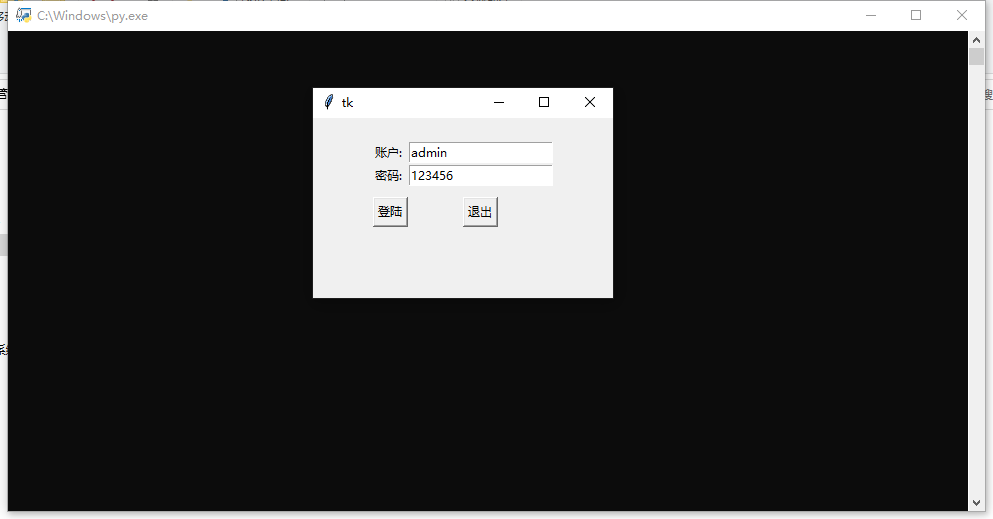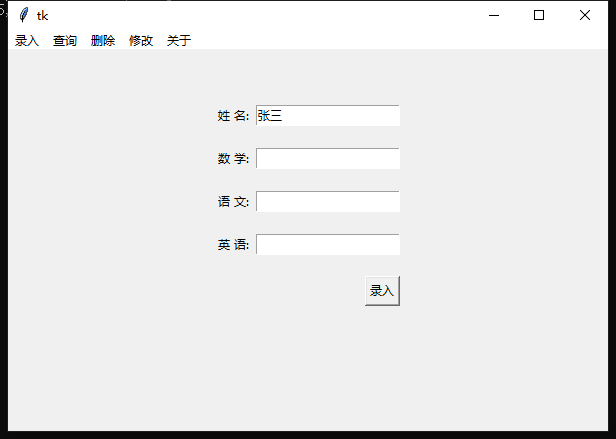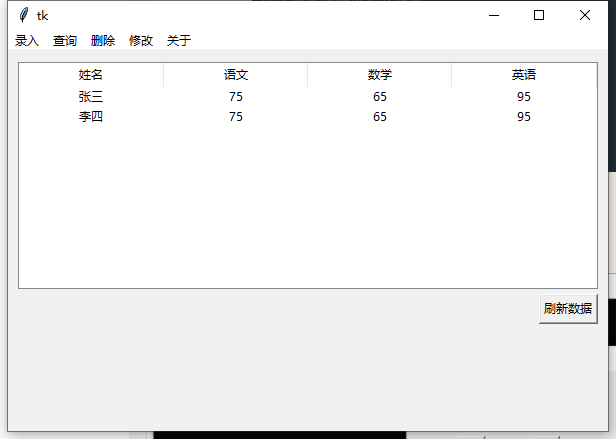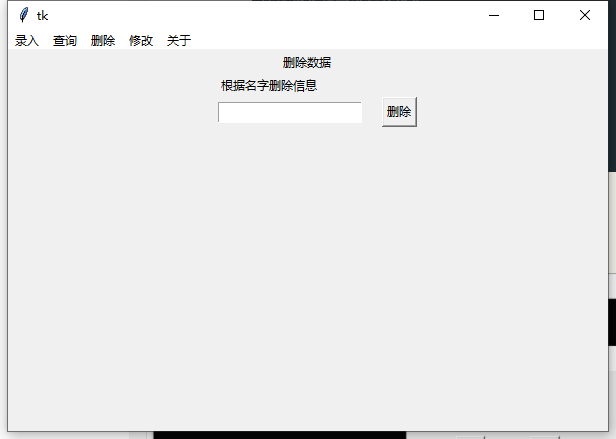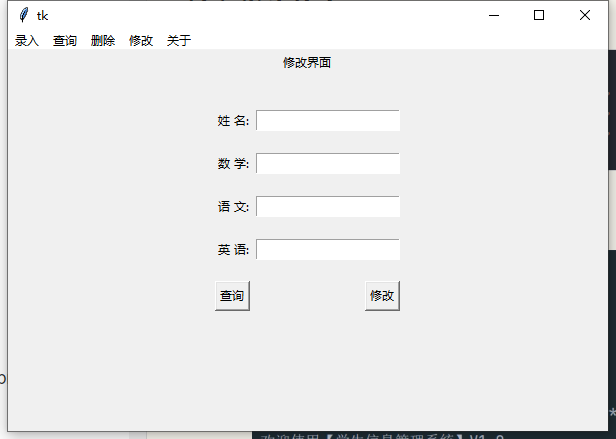Original: https://www.cnblogs.com/qshhl/p/15505292.html
Author: 松鼠爱吃饼干
Title: 只需用Python基础知识就能做的【学生信息管理系统】，学生党的福利，毕设、期末作业必备技能

## Title: Python 数据分析师的基本修养• 业界知识 -数据分析的基础就是为行业服务，足够的业界知识能让数据分析师了解究竟哪些数据才能为行业提供更深入的洞察
• 编程技巧 -数据分析师需要清楚应该使用哪些库来简化和处理数据，进而从中找到所需的资料
• 数据分析 -除了本身的数据分析能力，数据分析师也需要懂得借助工具来提取数据中的价值
• 可视化技能 -只是提取数据是不够的，数据分析师需要把这些数据整理好后进行可视化，总结并呈现给他人

## 分析数据## 读取数据

``````import pandas as pdfrom tabulate import tabulatedf = pd.read_csv('diabetes.csv'
``````

``````print(tabulate(df, headers = 'keys', tablefmt = 'psql'))
``````## 数据可视化Original: https://blog.51cto.com/u_15340774/5547329
Author: TeamCode
Title: Python 数据分析师的基本修养